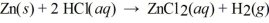/
/
/
121) The action of some commercial drain cleaners based the
Not my Question
Flag Content

# Question : 121) The action of some commercial drain cleaners based the : 1403242

121) The action of some commercial drain cleaners is based on the following reaction:

2 NaOH(s) + 2 Al(s) + 6 H2O(l)  →  2 NaAl(OH)4(s) + 3 H2(g)

What is the volume of H2 gas formed at STP when 6.32 g of Al reacts with excess NaOH?

A) 3.50 L

B) 5.25 L

C) 7.87 L

D) 8.59 L

122) How many liters of oxygen are needed to exactly react with 19.8 g of methane at STP?

CH4(g) + 2 O2(g)  →  CO2(g) + 2 H2O(l)

A) 13.9 L

B) 27.8 L

C) 55.5 L

D) 60.5 L

123) When 14.0 g of zinc metal reacts with excess HCl, how many liters of H2 gas are produced at STP?

A) 0.208 L

B) 0.416 L

C) 4.80 L

D) 9.60 L

124) How many grams of XeF6 are required to react with 0.579 L of hydrogen gas at 6.46 atm and 45°C in the reaction shown below?

XeF6(s) + 3 H2(g)  →  Xe(g) + 6 HF(g)

A) 9.58 g

B) 11.7 g

C) 35.2 g

D) 106 g

125) A 1.000 kg sample of nitroglycerine, C3H5N3O9, explodes and releases gases with a temperature of 1985°C at 1.100 atm. What is the volume of gas produced?

4 C3H5N3O9(s)  →  12 CO2(g) + 10 H2O(g) + 6 N2(g) + O2(g)

A) 742.2 L

B) 3525 L

C) 4730 L

D) 5378 L

126) Calcium hydride (CaH2) reacts with water to form hydrogen gas:

CaH2(s) + 2H2O(l)  →  Ca(OH)2(aq) + 2 H2(g)

How many grams of CaH2 are needed to generate 48.0 L of H2 gas at a pressure of 0.888 atm and a temperature of 32°C?

A) 50.7

B) 0.851

C) 143

D) 35.8

E) 71.7

127) How many grams of zinc metal are required to produce 2.00 liters of hydrogen gas at STP according to the chemical equation shown below?A) 0.171 g

B) 5.83 g

C) 11.7 g

D) 131 g

128) How many grams of calcium hydride are required to produce 4.56 L of hydrogen gas at 25.0°C and 0.975 atm pressure according to the chemical equation shown below?

CaH2(s) + 2 H2O(l)  →  Ca(OH)2(aq) + 2 H2(g)

A) 3.82 g

B) 7.64 g

C) 15.3 g

D) 45.6 g

129) How many liters of hydrogen gas can be generated by reacting 9.25 grams of barium hydride with water at 20°C and 755 mm Hg pressure according to the chemical equation shown below?

BaH2(s) + 2 H2O(l)  →  Ba(OH)2(aq) + 2 H2(g)

A) 0.219 L

B) 0.799 L

C) 1.60 L

D) 3.21 L

130) How many grams of water are required to produce 5.50 L of hydrogen gas at 25.0°C and 755 mm Hg pressure according to the chemical equation shown below?

BaH2(s) + 2 H2O(l)  →  Ba(OH)2(aq) + 2 H2(g)

A) 2.01 g

B) 4.02 g

C) 4.07 g

D) 8.04 g

## Solution 5 (1 Ratings )

Solved
Chemistry 1 Year Ago 43 Views